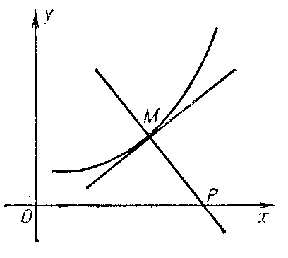# Ribaucour curve

(diff) ← Older revision | Latest revision (diff) | Newer revision → (diff)

A planar curve with curvature radius $R$ at an arbitrary point $M$ proportional to the length of the segment of the normal $MP$ (see Fig.).Figure: r081760a

The equation for the Ribaucour curve in Cartesian orthogonal coordinates is

$$x=\int\limits_0^y\frac{dy}{\sqrt{(y/c)^{2n}-1}},$$

where $n=MP/R$. If $n=1/h$ ($h$ is any integer), then a parametric equation for the Ribaucour curve is

$$x=(m+1)C\int\limits_0^t\sin^{m+1}tdt,\quad y=C\sin^{m+1}t,$$

where $m=-(n+1)n$. When $m=0$, the Ribaucour curve is a circle; when $m=1$, it is a cycloid; when $m=-2$, it is a catenary; and when $m=-3$, it is a parabola.

The length of an arc of the curve is

$$l=(m+1)C\int\limits_0^t\sin^mtdt;$$

and the curvature radius is

$$R=-(m+1)C\sin^mt.$$

This curve was studied by A. Ribaucour in 1880.

How to Cite This Entry:
Ribaucour curve. Encyclopedia of Mathematics. URL: http://encyclopediaofmath.org/index.php?title=Ribaucour_curve&oldid=36400
This article was adapted from an original article by D.D. Sokolov (originator), which appeared in Encyclopedia of Mathematics - ISBN 1402006098. See original article